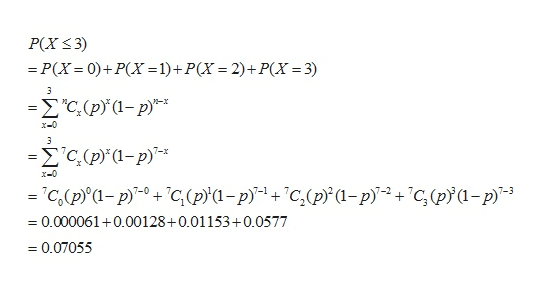# In a survey of 3 of 4 students said the courts show too much concern for criminals.  Find the probability that at most 3 out of 7 randomly selected students will agree with this statement.

Question

In a survey of 3 of 4 students said the courts show too much concern for criminals.  Find the probability that at most 3 out of 7 randomly selected students will agree with this statement.

check_circle

Step 1

According to the given question, in a survey of 3 of 4 students said the courts show too much concern for criminals.

Therefore the probability of success is defined as:

Step 2

Let us X be the random variable shows that the number of students will agree with this statement.

Therefore X follows binomial distribution with n=7 and p=0.75 or,

Step 3

Therefore the probability that at most 3 out of 7 randomly sele...help_outlineImage TranscriptioncloseР(X < 3) — Р(X - 0)+ P(X -1) + P(X — 2)+Р(X — 3) "C,(p(-p х-с -2С (p'1-p)* х-0 -С.(р'1- р)" + "с, (р'а - р)"* +"с,(p'а-р)* +"ҫ,(pia-p 0.000061 0.00128+0.01153+0.0577 7-0 7-3 0.07055 _ fullscreen

### Want to see the full answer?

See Solution

#### Want to see this answer and more?

Solutions are written by subject experts who are available 24/7. Questions are typically answered within 1 hour.*

See Solution
*Response times may vary by subject and question.
Tagged in

### Statistics